• 时间复杂度是同一问题可用不同算法解决，而一个算法的质量优劣将影响到算法乃至程序的效率。算法分析的目的在于选择合适算法和改进算法。 定义： 在计算机科学中，算法的时间复杂度是一个函数，它定性描述了该算法...
时间复杂度是同一问题可用不同算法解决，而一个算法的质量优劣将影响到算法乃至程序的效率。算法分析的目的在于选择合适算法和改进算法。

定义：

在计算机科学中，算法的时间复杂度是一个函数，它定性描述了该算法的运行时间。这是一个关于代表算法输入值的字符串的长度的函数。时间复杂度常用大O符号表述，不包括这个函数的低阶项和首项系数。

算法复杂度分为时间复杂度和空间复杂度。其作用： 时间复杂度是指执行算法所需要的计算工作量；而空间复杂度是指执行这个算法所需要的内存空间。（算法的复杂性体现在运行该算法时的计算机所需资源的多少上，计算机资源最重要的是时间和空间（即寄存器）资源，因此复杂度分为时间和空间复杂度）。

计算方法

1.一般情况下，算法中基本操作重复执行的次数是问题规模n的某个函数，用T(n)表示，若有某个辅助函数f(n),使得当n趋近于无穷大时，T(n)/f(n)的极限值为不等于零的常数，则称f(n)是T(n)的同数量级函数。记作T(n)=O(f(n)),称O(f(n)) 为算法的渐进时间复杂度，简称时间复杂度。

分析：随着模块n的增大，算法执行的时间的增长率和 f(n) 的增长率成正比，所以 f(n) 越小，算法的时间复杂度越低，算法的效率越高。

在计算时间复杂度的时候，先找出算法的基本操作，然后根据相应的各语句确定它的执行次数，再找出 T(n) 的同数量级（它的同数量级有以下：1，log2n，n，n log2n ，n的平方，n的三次方，2的n次方，n!），找出后，f(n) = 该数量级，若 T(n)/f(n) 求极限可得到一常数c，则时间复杂度T(n) = O(f(n))

例：算法


for(i=1; i<=n; ++i)
{
for(j=1; j<=n; ++j)
{
c[i][j] = 0;//该步骤属于基本操作执行次数：n的平方次
for(k=1; k<=n; ++k)
c[i][j] += a[i][k] * b[k][j];//该步骤属于基本操作执行次数：n的三次方次
}
}

则有   ，根据上面括号里的同数量级，我们可以确定 n的三次方 为T（n）的同数量级
则有  ，然后根据 T(n)/f(n) 求极限可得到常数c
则该算法的时间复杂度：T(n) = O(n^3) 注：n^3即是n的3次方。

该计算差不多将每一步的时间复杂度加起来 取最高次项的那一个 去掉前面的数

例：
(2n^3)/2+3n+4n^2
则其取最高次项(2n^3)/2 由于前面的数对结果影响很小 顾可以舍去
则其时间复杂度为O(n^3)

第一次写博客写的水平比较低  百度百科里借鉴了一下 可能存在一些瑕疵。
展开全文• 数据结构时间复杂度
（1）算法O（n），关注n的阶数，当数十分大的时候，常数可以忽略。O（n）又称为大O记法。
（2）T（n）=O（f（n）），随着n变化而变化，f（n）是某个函数，执行的次数等于时间，一般情况下，T（n）增长最慢的算法最优。
（3）3个求和算法时间复杂度：O（1），O（n），O（n^2）甚至更多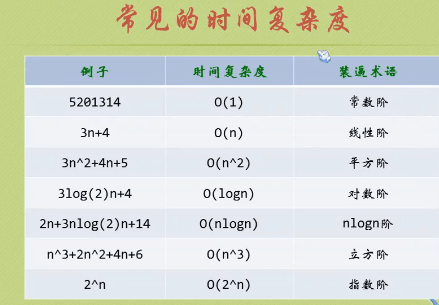（4）推到O（n）:
1,，用1取代时间中所有加法常数（哪些可以忽略）。
2，在修改后的运行函数中，只保留最高项。
3，如果最高项存在且不是1，去除这个最高项相乘的常数。例如：3n^3  变成n^3，n的三次方。
4,得到最后结果是大O阶

（一）常数阶：
例如：
System.out.println("m 转换为String型后与整数20的求和结果为： "+(str1+20));System.out.println("str2和str3转换成字符串后相加结果: "+(str2+str3));
时间复杂度是：O（1）而不是O（2），只关注与n有关的。所有的加法常数，只给O（1）就可以了。

（二）线性阶
for(int j=0;j<n;j++){System.out.println("m 转换为String型后与整数20的求和结果为： "+(str1+20)); }
时间复杂度是：O（n）执行了n次，如果是100,则是100次，n=1000则是1000次

（三）平方阶
例如：n=100,外层执行一次，内层执行100次，两个循环则是100*100
for(int i=0;i<100;i++){for(int j=0;j<100;j++)System.out.print("下标【"+i+"】:"+number[i]+" ");}
因此，时间复杂度是：O（n^2），如果是三个嵌套循环，则是O（n^3）。

例如：
for(int i=0;i<n;i++){for(int j=i;i<n;j++)System.out.print("下标【"+i+"】:"+number[i]+" ");}
当外层i=0，n=100则内层循环执行n次，当i=1时候，内存循环执行n-1次。
于是：n+(n-1)+(n-2)+...+1=n(n+1)/2.高斯先生算法。=n^2/2+n/2,根据大O推算，时间复杂度是：O（n^2），只算最高阶。

（四）对数阶
2^x=n,得出：x=log(2)n,x是循环的次数，log 2为底，小写n）因此时间复杂度是：O（logn)

空间复杂度：
S（n）=O（f（n）），f（n）为语句关于n所占存储空间的函数。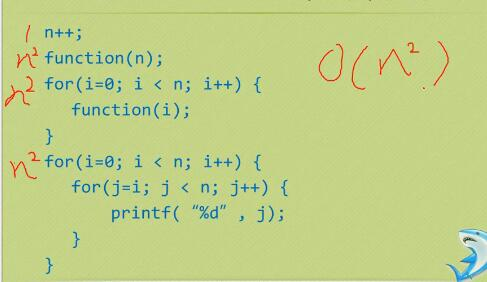展开全文算法 函数
• ## 数据结构时间复杂度题库

万次阅读 多人点赞 2018-10-16 10:39:52
1、下列说法正确的是： A、如果函数f(n)是O(g(n))，g(n)是O(h(n))，那么f(n)是O(h(n)) 解析： B、如果函数f(n)是O(g(n))，g(n)是O(h(n))，那么f(n)+g(n)是O(h(n)) 解析： ...logan=...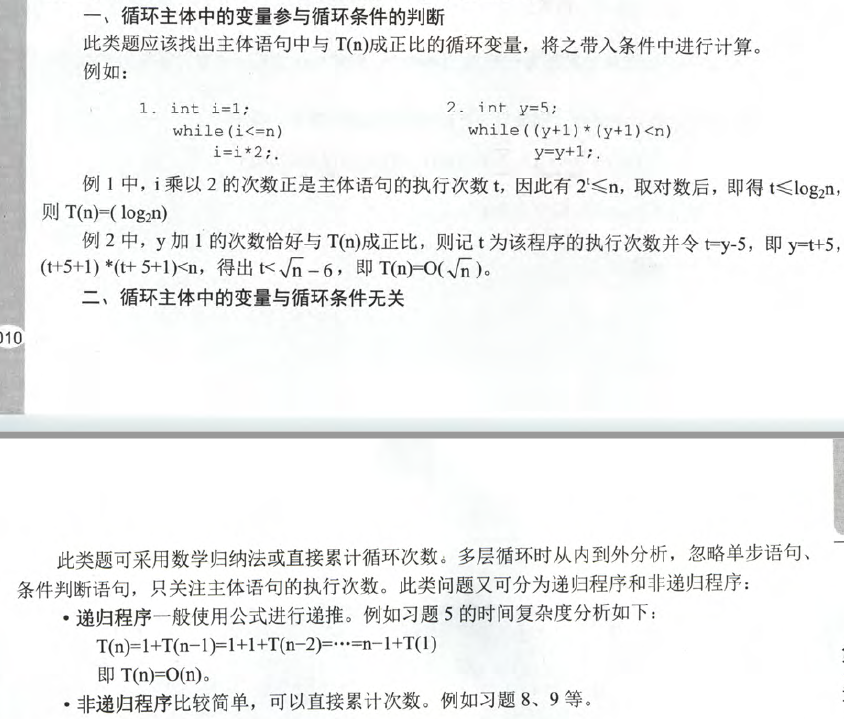1、下列说法正确的是：

A、如果函数f(n)是O(g(n))，g(n)是O(h(n))，那么f(n)是O(h(n))

解析：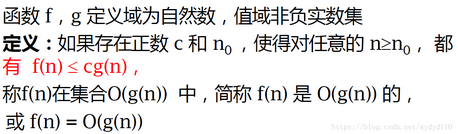B、如果函数f(n)是O(g(n))，g(n)是O(h(n))，那么f(n)+g(n)是O(h(n))

解析：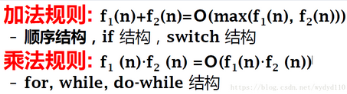C、如果a>b>1,logan是O(logbn)，但logbn不一定是O(logan)

解析：

logan=log(n)/log(a),logbn=log(n)/log(b),所以前者与后者只差了一个常数项，所以logbn一定是O(logan)

x=logaN，a为底数       log2(8) = log8/log2=3

D、函数f(n)是O(g(n))，当常数a足够大时，一定有函数g(n)是O(af(n))

解析：当f(n)=n，g(n)=n*2=n*n， af(n)=a*n，无论a多大，g(n)都不可能是O(af(n))

2、已知一个数组a的长度为n，求问下面这段代码的时间复杂度:

for (i=0,length=1;i<n-1;i++){

for (j = i+1;j<n && a[j-1]<=a[j];j++)   //a[j-1]<=a[j]有序

if(length<j-i+1)

length=j-i+1;

}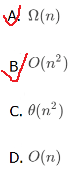解析

实际上是求a中有序子数组中最长的长度。譬如，在[1, 8, 1, 2, 5, 0, 11, 9]中，最长的是[1, 2, 5]，长度为3 。其时间复杂度与a中元素的实际取值状态相关。 1)若a的所有元素是按照降序方式排列。则外层循环n-1次，每次内层只执行一次，整个开销为θ(n) 2)若a的所有元素是按照升序方式排列。则外层循环n-1次，每次内层需要执行n-i-1次，整个开销为θ(n^2) 所以，一般来说，时间复杂度是Ω(n)的，也是O(n^2)

3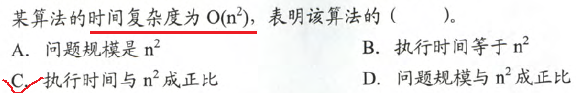4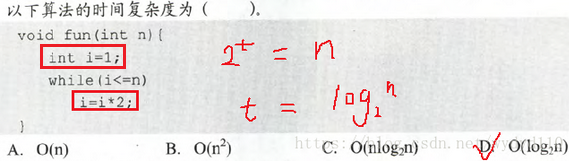解析：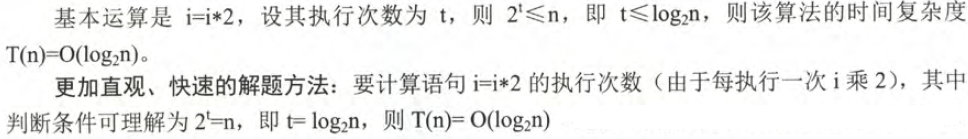5、2011年计算机联考真题（重做总结一遍）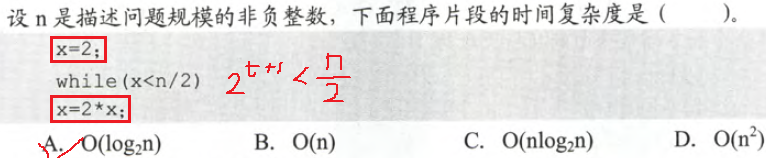解析：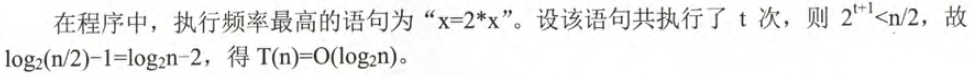6、2012年计算机联考真题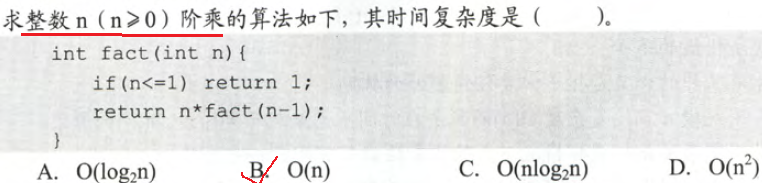解析：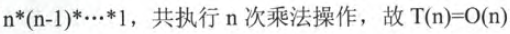7、2013年计算机联考真题（重做总结一遍）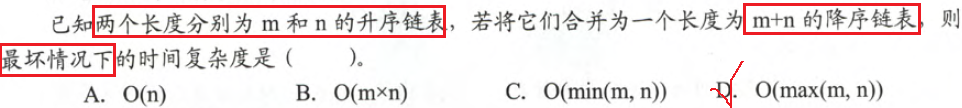解析：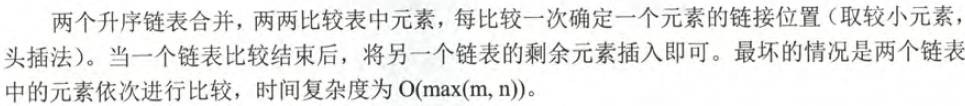8、2014年计算机联考真题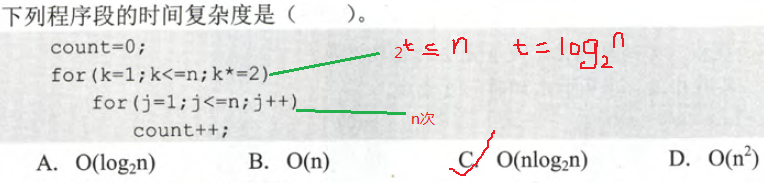9、2017年计算机联考真题（以后重做总结一遍）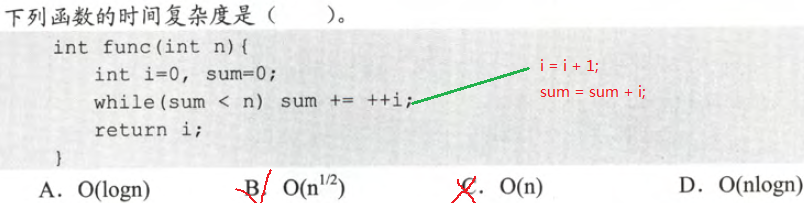解析：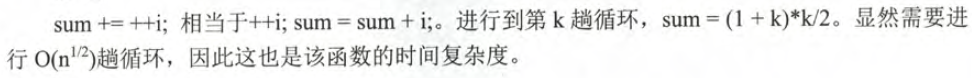10、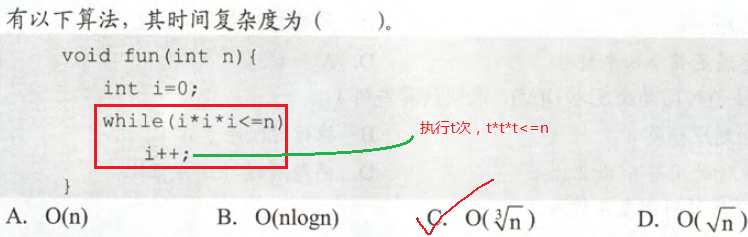解析：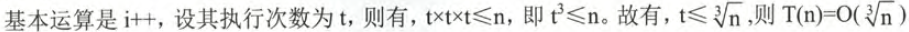11（以后重做总结一遍）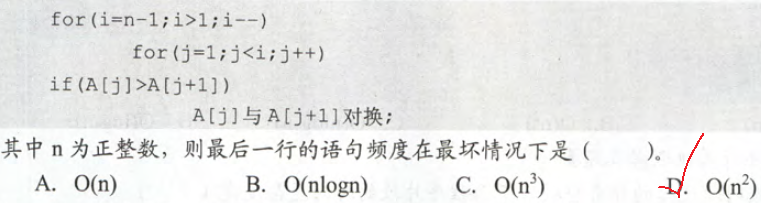解析：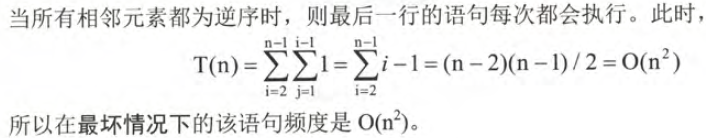12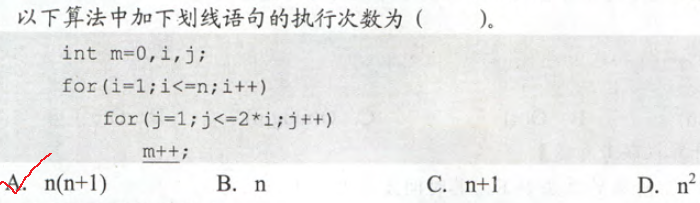解析：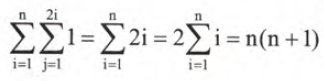展开全文• 数据结构时间复杂度分析 由于疫情原因，没办法去学校，数据结构也是看了很多遍，但知识点还是很容易忘，所以就用博客的方式来记录。 对于一般算法的时间复杂度，一般直接找到它的循环的次数即可，例： if ( A > B...
数据结构时间复杂度分析
由于疫情原因，没办法去学校，数据结构也是看了很多遍，但知识点还是很容易忘，所以就用博客的方式来记录。
对于一般算法的时间复杂度，一般直接找到它的循环的次数即可，例：
if ( A > B ) {
for ( i=0; i<N; i++ )
for ( j=N*N; j>i; j-- )
A += B;
}
else {
for ( i=0; i<N*2; i++ )
for ( j=N*2; j>i; j-- )
A += B;
}

对（A>B）情况，最外层运行n-1次，内层运行最大运行次数为n²-1（当i=0）时，所以O（n）=n³。
对（A<=B）情况，最外运行2n-1次，内层运行最大运行次数为2n-1（当i=0）时，O（n）=n²。
对于总的时间复杂度O（n）应取最大的时间复杂度，即O（n）=n³。
然后是递归算法的时间复杂度，一般列出该算法各种情况下，运行次数，最后根据题意对应列出式子，例：
void fun (int a[],int n,int k)
{
int i;
if(k==n-1)
for(i=0;i<n;i++)
print("%d\n",a[i]);
else
{
for (i=k;i<n;i++)
a[i]=a[i]+i*j;
fun(a,n,k+1);
}
}

求 fun(a,n,0),下面我给出解题格式
设fun(a,n,k)的时间为T1（n，k）,则 fun(a,n,0）=T1（n,0）
T(n,k)=

n    当k=n-1时；
（n-k）+T1（n，k+1）  其他情况

T（n,0）=n+T1(n,k1)
…
= n+n-1+…+2+T1(n,n-1)
=(n+2)(n-1)/2+n
可以看出该算法的时间复杂度为O（n）=n²
对于特别复杂的递归算法，我们可以用分而治之的方法，例：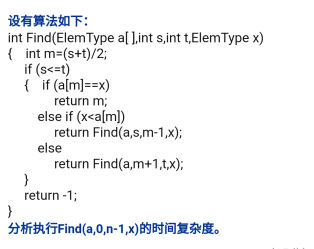从这里可以看出，要用之前做递归的方法，是特别复杂的。
分析代码我们可以得出，该算法是在数组中找到对应的值，并且用的是二分法，所以T（n）=T（n/2）+1;a=1（归约后我们可以看出，只需只要比较一半了，即子问题为1）；b=2（规模减半，即子问题为n/2）;f（n）=1（归约时，我们比较了1次，组合为自动0次，即总的工作量为1）
我们可以用主定理来求解，得到答案T（n）=O（log n），讲解可以看此处主定理，这里就不讲解了。


展开全文•链表 队列 算法
• 时间复杂度算法效率时间复杂度原理简单示例3.空间复杂度原理简单示例 1.什么是数据结构 数据结构（Data Structure）是一门研究数据的组织和管理的学科。往往从外在表现为一组数据的集合或者容器。 概念解释： 元素...java
• 算法效率 算法效率分析分为两种：第一种是时间效率，第二种是空间效率。时间效率被称为时间复杂度，而空间效率被...时间复杂度的定义：在计算机科学中，算法的时间复杂度是一个函数，它定量描述了该算法的运行时间。java
• 时间效率被称为时间复杂度,空间效率被称为空间复杂度.时间复杂度主要衡量的是一个算法的运行速度，而空间复杂度主要衡量一个算法所需要的额外空间，在计算机发展的早期，计算机的存储容量很小。所以对空间复杂度很是...
• 1：概念：时间复杂度是总运算次数表达式中受n的变化影响最大的那一项（不含系数） 5*n^5+10000000的时间复杂度是n^5 2：计算方法：时间复杂度就是一个算法中的语句执行次数最多的一个。 1.一个算法执行所耗费的...
• 怎么分析一段代码的时间复杂度呢？三个方法： 1. 只关注循环执行次数最多的一段代码 2. 加法法则，T(n) = O(max(f(n), g(n))) 3. 乘法法则，O(f(n)) * O(g(n)) = O(f(n) * g(n)) 只要算法中不存在循环，即使有...
• Hashmap基于hash表, 时间复杂度O(1)~O(N)之间 TreeMap 基于平衡二叉树, 时间复杂度O(Logn) 各种排序算法时间复杂度: 排序法 最差时间分析 平均时间复杂度 稳定度 空间复杂度 冒泡排序 O(n2) O(n2) 稳定 O(1)...java
• 时间复杂度 时间复杂度简单的理解就是执行语句的条数。如果有循环和递归，则忽略简单语句，直接算循环和递归的语句执行次数。 比如： [java] view plaincopy int x = 1...
• 图片来源： https://blog.csdn.net/ted_cs/article/details/82881831算法 1024程序员节
•  它的时间复杂度是多少？   自己计算了一下，数学公式忘得差不多了，郁闷； （1）时间复杂性是什么？ 时间复杂性就是原子操作数，最里面的循环每次执行j次，中间循环每次执行   a[i]=1+2+3+...+i=i*(i+1)/2...
• 好不容易找到的~超详细..相信看了之后都能战胜时间复杂度这个难题！
• 求O，时间复杂度：  算数级数：   幂方级数：比幂次高出一阶       几何级数（a&gt;1)：与末项同阶    收敛级数： 未必收敛，长度有限    ...复杂度 公式 数学
• 势能函 [公式] 将每个数据结构 D [公式] 映射到一个实数 [公式] ，此值即为关联到数据结构 [公式] 的势能，第 i 个操作的摊还代价 [公式] 用势函数 [公式] 定义为： [公式] 。因此，第每个操作的摊还代价等于操作的...
• 数据结构复杂度 在介绍复杂度之前我们现分享一个名词叫算法效率 算法效率：算法效率是指算法执行的时间，算法执行时间需通过依据该算法编制的程序在计算机上运行时所消耗的时间来度量。 算法效率也分为两种： 一种...
• 文章目录数据结构之复杂度衡量1、算法：2、算法构成的基本要素3、算法效率4、复杂度度量4.1 时间复杂度4.2 空间复杂度4.3 复杂度分析4.4 递归4.5 封装 学数据结构的主要原因： 刷Leetcode算法题，无奈做完第一道......

# 数据结构时间复杂度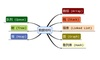数据结构 订阅